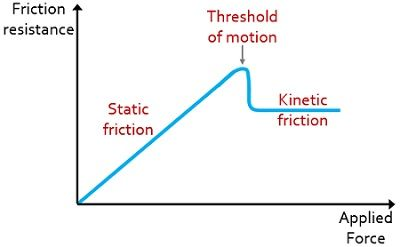# Difference Between Static and Kinetic Friction

Static and Kinetic are the two categories in which friction is classified. The significant difference between static and dynamic friction is that static friction is the frictional force acting on a body that is resting. As against kinetic friction is the force of friction acting on a body that is in a relative motion.

Thus, we can say, these two types of frictions are majorly differentiated on the basis that whether the force of friction is acting on the body at rest or in motion.

What is Friction?

The opposition experienced by an object to initiate motion or while in motion relative to a surface is known as friction. Unlike gravity or electromagnetism, the frictional force is not of fundamental nature. It acts opposite to the direction in which the object or body is trying to move or moving.

## Content: Static Vs Kinetic Friction

### Comparison Chart

Basis for ComparisonStatic FrictionKinetic Friction
BasicIt is associated with body at rest.It is associated with motion of object.
Magnitude MoreComparatively less
Denoted asFsFk
ExpressionμsFNμkFN
Magnitude of forceDependentIndependent
NatureIt is opposition to the beginning of motion.It is the opposition relative to motion of the body.
ValueCan be zero.Can never be zero.
BehaviourShows linear increase up to a maximum value.Shows invariable nature and remains constant.
When it actsIn the absence of relative motion.In the presence of relative motion.
ExamplePen placed on the table.Moving a pen over a table top.

### Definition of Static Friction

The force of friction which causes the bodies at rest to be at rest is known as static friction. More simply, due to static friction, the bodies remain at rest. It basically acts between two surfaces at rest.

The magnitude of the force of static friction can be zero and can range between 0 to the minimum value of the force that can cause the motion. Due to static friction, the body experiences resistance and this prevents the body to move down in the slopy region.

Static friction is regarded as a feature of a surface with irregularities that resist the motion of the object. To overcome this static friction, an external force is required that can move the object over the surface. The expression for the maximum force required to overcome static friction is the product of the coefficient of static friction(μs) and normal force. Thus, is given as:

Fs = μsFN

### Definition of Kinetic Friction

The resistance to the motion of a body over a surface is known as kinetic friction. We know that once the body overcomes static friction then it starts moving so, the force of friction that acts between moving surfaces is the kinetic friction. It is sometimes referred as dynamic friction.

The magnitude of kinetic friction can never be zero as some amount of force is necessarily be required to stop a body that is in motion. This force is applied in the opposite direction to the movement of the object.

The coefficient of kinetic friction is denoted as μk and it depends on both the surface in contact. It is to be noted here that μk is generally less than μs for the same materials. The expression for the maximum force required to overcome kinetic friction is the product of the coefficient of kinetic friction and normal force. Thus, is given as:

Fk = μkFN

## Key Differences Between Static and Dynamic Friction

1. The key factor of differentiation between static and kinetic friction is that the static friction acts on a body that is at rest. On the contrary, kinetic friction acts when there is a relative motion of two surfaces. We can make it more clear by understanding that an object when is stationary does not automatically glide over the surface, so the frictional force that keeps the object stable is the static friction. While, whenever, there is a movement of an object over a surface then the surface also applies some frictional force to it so as to retard the movement, this force of friction is kinetic friction.
2. The static friction shows a linear rise up to a maximum value when some external force is applied. While kinetic friction shows invariable nature and remains constant irrespective of the force applied.3. The magnitude of the coefficient of static friction is comparatively more than that of kinetic friction. Thus, the force of friction that is required to keep the object stable is obviously more than the frictional force which opposes the motion of the object.
4. Static friction is dependent on the magnitude of the force applied while kinetic friction is independent of the magnitude of the force which is applied.
5. Static friction corresponds to the opposition to start a motion whereas kinetic friction is the opposition offered to something which is already in motion.
6. The value of static friction can be zero but the value of kinetic friction can never be zero.
7. The various examples of static friction are a pen placed on the tabletop, a chair on a floor, a stationary vehicle on the road, etc. However, some examples of kinetic friction are sliding the box on the floor, movement of the vehicle on road, writing anything on the blackboard with chalk.

### Conclusion

So, the above discussion concludes that static and kinetic friction are two different but interrelated terminologies. Static friction comes into action whenever there is an absence of relative motion of objects. But kinetic friction is active in the presence of relative motion of objects.